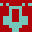# matmul

#### FP matrix multiplication

```\ matrix muliplication benchmark

[undefined] {: [if]
[undefined] parse-name [if]
s" extensions/parse-name.fs" included
[then]
s" extensions/locals.fs" included
[then]

: init-matrix {: m ncols nrows nstart -- :}
\ initialize m with floats nstart, nstart+1, ...
nstart nrows 0 ?do
ncols 0 ?do
dup 0 d>f m j ncols * i + floats + f! 1+
loop
loop
drop ;

500 constant dim
dim dup * floats allocate throw constant a a dim dim     1 init-matrix
dim dup * floats allocate throw constant b b dim dim 30000 init-matrix
dim dup * floats allocate throw constant c

a b c dim dim dim matmulr

fvariable sum 0. d>f sum f!

: checksum ( m ncols nrows -- x )
* 0 ?do ( m1 )
dup f@ sum f@ f+ sum f! float+ loop
drop ;

[defined] f~ [if]
c dim dup checksum sum f@ 2423178953093560000. d>f -1e-5 f~ 0= [if]
.( result incorrect ) cr
[else]
.( result correct) cr
[then]
[then]
```

## by AntonErtl